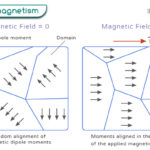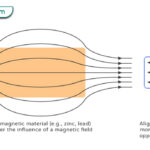Home / Physics / Unbalanced Force

# Unbalanced Force

A force is a physical entity that is applied to an object in order to displace it. The ultimate effect is a ‘push’ or ‘pull’ that the object experiences. A force is a vector that has both magnitude and direction.

## What is Unbalanced Force

Unbalanced forces refer to a set of forces acting on an object such they affect its motion. When two unequal forces act on an object from opposite sides, there is a net force, called the resultant force. The effect of this force is to either displace or tilt an object. When an unbalanced force is acting on a moving object, it changes the direction or speed.

What happens when an unbalanced force acts on an object?

Here are some facts and characteristics of the unbalanced force. When an unbalanced force acts on an object, the following can happen:

• Causes change in an object’s motion: A stationary object moves with acceleration. A moving object changes its direction or speed or comes to a stop. The motion obeys Newton’s law.
• The applied forces are added when they are in the same direction and subtracted when they are opposite.
• The sum of all forces acting on the object along a line is never equal to zero.

## Examples of Unbalanced Force

Here are a few examples of the unbalanced force.

• A free-falling object under the influence of gravity
• Two people of unequal weight on a see-saw
• A force applied to a book lying on a table such that it overcomes friction and accelerates
• A tug-of-war game where one team applies more force and wins

## Unbalanced Force Equation

Suppose F1 and F2 are two unequal forces acting on an object in opposite directions. Then, by definition of an unbalanced force, the net force acting on the object is non-zero.

Unbalanced Force Formula: F1 – F2 0

For example, a book of mass m is lying on a table. An externally applied force FA acts on it. It overcomes the friction FK and moves with an acceleration a. According to Newton’s second law, the force experienced by the book is given by

ma = FA – FK

As can be seen, the result of unbalanced forces is to accelerate the object.

## FAQ

Q.1. Is friction an unbalanced force?

Ans. It depends upon whether the friction force balances the applied force or not. In static friction, the object remains motionless because the static friction force balances the applied force. In kinetic friction, the object moves against the kinetic friction force in the applied force’s direction. If the object moves with a constant velocity, then the two forces are balanced. If the object moves with acceleration, then the two forces are unbalanced.

Article was last reviewed on Thursday, February 2, 2023

### Related articlesWave-Particle DualityPhotoelectric EffectFerromagnetismDiamagnetism